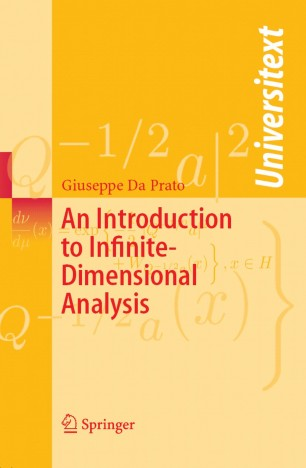# An Introduction to Infinite-Dimensional Analysis

• Giuseppe Da PratoTextbook

1. Front Matter
Pages i-x
2. Pages 1-24
3. Pages 25-33
4. Pages 35-49
5. Pages 69-82
6. Pages 83-92
7. Pages 93-108
8. Pages 109-123
9. Pages 125-135
10. Pages 137-163
11. Pages 165-191
12. Back Matter
Pages 193-213

### Introduction

In this revised and extended version of his course notes from a 1-year course at Scuola Normale Superiore, Pisa, the author provides an introduction – for an audience knowing basic functional analysis and measure theory but not necessarily probability theory – to analysis in a separable Hilbert space of infinite dimension.

Starting from the definition of Gaussian measures in Hilbert spaces, concepts such as the Cameron-Martin formula, Brownian motion and Wiener integral are introduced in a simple way. These concepts are then used to illustrate some basic stochastic dynamical systems (including dissipative nonlinearities) and Markov semi-groups, paying special attention to their long-time behavior: ergodicity, invariant measure. Here fundamental results like the theorems of  Prokhorov, Von Neumann, Krylov-Bogoliubov and Khas'minski are proved. The last chapter is devoted to gradient systems and their asymptotic behavior.

### Keywords

Brownian motion Gaussian measures Hilbert space Markov processes Probability theory Sobolev space White noise functional analysis invariant measures measure theory

#### Authors and affiliations

• Giuseppe Da Prato
• 1
1. 1.Scuola Normale SuperiorePisaItaly